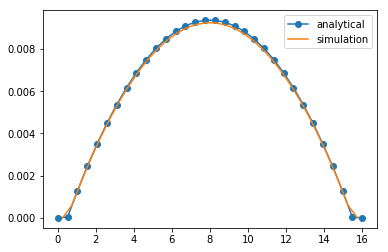# Tutorial 4 : The lattice-Boltzmann method in ESPResSo - Part 4¶

### 6 Poiseuille flow in ESPResSo¶

Poiseuille flow is the flow through a pipe or (in our case) a slit under a homogeneous force density, e.g. gravity. In the limit of small Reynolds numbers, the flow can be described with the Stokes equation. We assume the slit being infinitely extended in $y$ and $z$ direction and a force density $f_y$ on the fluid in $y$ direction. No slip-boundary conditions (i.e. $\vec{u}=0$) are located at $x = \pm h/2$. Assuming invariance in $y$ and $z$ direction and a steady state, the Stokes equation is simplified to:

\begin{equation} \mu \partial_x^2 u_y = f_y \end{equation}

where $f_y$ denotes the force density and $\mu$ the dynamic viscosity. This can be integrated twice and the integration constants are chosen so that $u_y=0$ at $x = \pm h/2$ to obtain the solution to the planar Poiseuille flow :

\begin{equation} u_y(x) = \frac{f_y}{2\mu} \left(h^2/4-x^2\right) \end{equation}

We will simulate a planar Poiseuille flow using a square box, two walls with normal vectors $\left(\pm 1, 0, 0 \right)$, and an external force density applied to every node.

Use the data to fit a parabolic function. Can you confirm the analytic solution?

In :
import logging
import sys

import numpy as np

import espressomd
import espressomd.lb
import espressomd.lbboundaries
import espressomd.shapes

logging.basicConfig(level=logging.INFO, stream=sys.stdout)

espressomd.assert_features(['LB_BOUNDARIES_GPU'])

# System constants
BOX_L = 16.0
AGRID = 0.5
VISCOSITY = 2.0
FORCE_DENSITY = [0.0, 0.001, 0.0]
DENSITY = 1.5
TIME_STEP = 0.01

system = espressomd.System(box_l=[BOX_L]*3)
system.time_step = TIME_STEP
system.cell_system.skin = 0.4

lbf = espressomd.lb.LBFluidGPU(agrid=AGRID, dens=DENSITY, visc=VISCOSITY, tau=TIME_STEP,
ext_force_density=FORCE_DENSITY)

logging.info("Setup LB boundaries.")
WALL_OFFSET = AGRID
top_wall = espressomd.lbboundaries.LBBoundary(shape=espressomd.shapes.Wall(normal=[1, 0, 0], dist=WALL_OFFSET))
bottom_wall = espressomd.lbboundaries.LBBoundary(shape=espressomd.shapes.Wall(normal=[-1, 0, 0], dist=-(BOX_L - WALL_OFFSET)))

logging.info("Iterate until the flow profile converges (5000 LB updates).")
system.integrator.run(5000)

logging.info("Extract fluid velocity along the x-axis")
fluid_velocities = np.zeros(lbf.shape)
for x in range(lbf.shape):
# Average over the nodes in y direction
v_tmp = np.zeros(lbf.shape)
for y in range(lbf.shape):
v_tmp[y] = lbf[x, y, 0].velocity
fluid_velocities[x] = np.average(v_tmp)

INFO:root:Setup LB boundaries.
INFO:root:Iterate until the flow profile converges (5000 LB updates).
INFO:root:Extract fluid velocity along the x-axis


The solution is available at /doc/tutorials/04-lattice_boltzmann/scripts/04-lattice_boltzmann_part4_solution.py

 S. Succi. The lattice Boltzmann equation for fluid dynamics and beyond. Clarendon Press, Oxford, 2001.
 B. Dünweg and A. J. C. Ladd. Advanced Computer Simulation Approaches for Soft Matter Sciences III, chapter II, pages 89–166. Springer, 2009.
 B. Dünweg, U. Schiller, and A.J.C. Ladd. Statistical mechanics of the fluctuating lattice-Boltzmann equation. Phys. Rev. E, 76:36704, 2007.
 P. G. de Gennes. Scaling Concepts in Polymer Physics. Cornell University Press, Ithaca, NY, 1979.
 M. Doi. Introduction to Polymer Physics. Clarendon Press, Oxford, 1996.
 Michael Rubinstein and Ralph H. Colby. Polymer Physics. Oxford University Press, Oxford, UK, 2003.
 Daan Frenkel and Berend Smit. Understanding Molecular Simulation. Academic Press, San Diego, second edition, 2002.
 W. E. Langlois and M. O. Deville. Exact Solutions to the Equations of Viscous Flow. In: Slow Viscous Flow, Springer, Cham, 2014.

Solution from scripts/04-lattice_boltzmann_part4_solution_cut.py

In :
import matplotlib.pyplot as plt

In :
# Extract fluid velocity along the x-axis
fluid_velocities = np.zeros((lbf.shape, 2))
for x in range(lbf.shape):
# Average over the node in y direction
v_tmp = np.zeros(lbf.shape)
for y in range(lbf.shape):
v_tmp[y] = lbf[x, y, 0].velocity
fluid_velocities[x, 0] = (x + 0.5) * AGRID
fluid_velocities[x, 1] = np.average(v_tmp)

def poiseuille_flow(x, force_density, dynamic_viscosity, height):
return force_density / (2.0 * dynamic_viscosity) * \
(height**2.0 / 4.0 - x**2.0)

# Note that the LB viscosity is not the dynamic viscosity but the
# kinematic viscosity (mu=LB_viscosity * density)
x_values = np.linspace(0.0, BOX_L, lbf.shape)
HEIGHT = BOX_L - 2.0 * AGRID
# analytical curve
y_values = poiseuille_flow(x_values - (HEIGHT / 2.0 + AGRID), FORCE_DENSITY,
VISCOSITY * DENSITY, HEIGHT)
# velocity is zero outside the walls
y_values[np.nonzero(x_values < WALL_OFFSET)] = 0.0
y_values[np.nonzero(x_values > BOX_L - WALL_OFFSET)] = 0.0

plt.plot(x_values, y_values, 'o-', label='analytical')
plt.plot(fluid_velocities[:, 0], fluid_velocities[:, 1], label='simulation')
plt.legend()
plt.show()